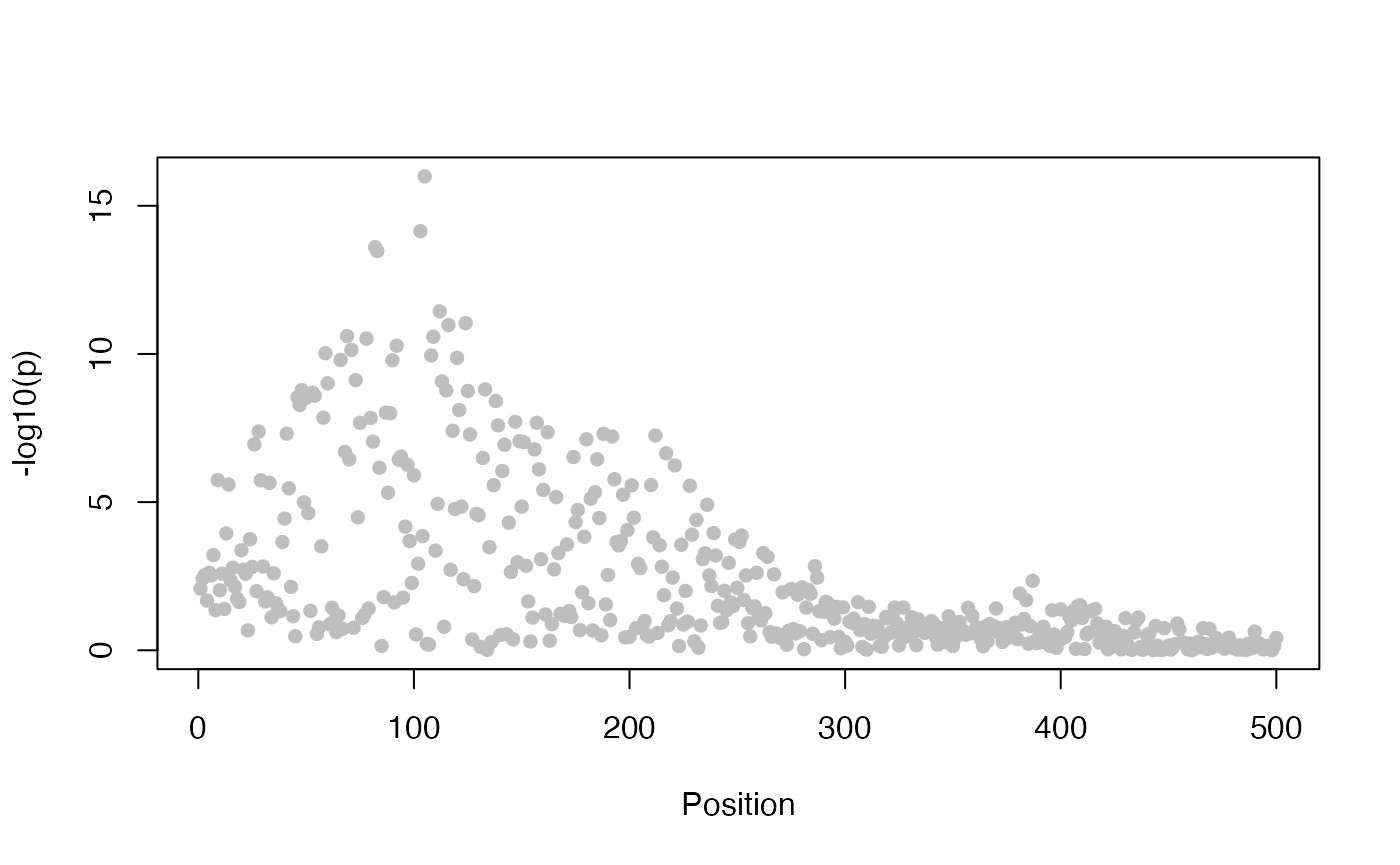# Fine mapping under a single causal variant assumption

The Approximate Bayes Factor colocalisation analysis described in the next section essentially works by fine mapping each trait under a single causal variant assumption and then integrating over those two posterior distributions to calculate probabilities that those variants are shared. Of course, this means we can look at each trait on its own quite simply, and we can do that with the function finemap.abf.

First we load some simulated data. See the data vignette to understand how to format your datasets.

library(coloc)
## This is a new update to coloc.
data(coloc_test_data)
attach(coloc_test_data)

Then we analyse the statistics from a single study, asking about the evidence that each SNP in turn is solely causal for any association signal we see. As we might expect, that evidence is maximised at the SNP with the smallest p value

plot_dataset(D1)my.res <- finemap.abf(dataset=D1)
my.res[21:30,]
##              V.       z.        r.       lABF. snp position prior       SNP.PP
## s21 0.013574490 2.210771 0.6760490  1.08851608 s21       21 1e-04 6.993866e-06
## s22 0.022519401 2.832099 0.5571217  1.82704569 s22       22 1e-04 1.463715e-05
## s23 0.013008412 2.381939 0.6853070  1.36601147 s23       23 1e-04 9.230645e-06
## s24 0.024858631 3.177590 0.5326188  2.30864180 s24       24 1e-04 2.369252e-05
## s25 0.005031597 1.470717 0.8491729 -0.02742696 s25       25 1e-04 2.291234e-06
## s26 0.027329180 3.027380 0.5089767  1.97676103 s26       26 1e-04 1.700111e-05
## s27 0.029092316 2.833849 0.4933483  1.64100022 s27       27 1e-04 1.215229e-05
## s28 0.012057181 2.079938 0.7014486  0.91287665 s28       28 1e-04 5.867297e-06
## s29 0.009216961 1.496951 0.7545115  0.14312596 s29       29 1e-04 2.717313e-06
## s30 0.007073637 1.725572 0.8001914  0.38612656 s30       30 1e-04 3.464762e-06

The SNP.PP column shows the posterior probability that exactly that SNP is causal. Note the last line in this data.frame does not correspond to a SNP, but to the null model, that no SNP is causal.

tail(my.res,3)

Finally, if you do have full genotype data as here, while this is a fast method for fine mapping, it can be sensible to consider multiple causal variant models too. One package that allows you to do this is GUESSFM, described in5

# (Approximate) Bayes Factor colocalisation analyses

## Introduction

The idea behind the ABF analysis is that the association of each trait with SNPs in a region may be summarised by a vector of 0s and at most a single 1, with the 1 indicating the causal SNP (so, assuming a single causal SNP for each trait). The posterior probability of each possible configuration can be calculated and so, crucially, can the posterior probabilities that the traits share their configurations. This allows us to estimate the support for the following cases:

• $$H_0$$: neither trait has a genetic association in the region
• $$H_1$$: only trait 1 has a genetic association in the region
• $$H_2$$: only trait 2 has a genetic association in the region
• $$H_3$$: both traits are associated, but with different causal variants
• $$H_4$$: both traits are associated and share a single causal variant

## The basic coloc.abf function

The function coloc.abf is ideally suited to the case when only summary data are available.

my.res <- coloc.abf(dataset1=D1,
dataset2=D2)
## PP.H0.abf PP.H1.abf PP.H2.abf PP.H3.abf PP.H4.abf
##  1.73e-08  7.16e-07  2.61e-05  8.20e-05  1.00e+00
##  "PP abf for shared variant: 100%"
print(my.res) 
## Coloc analysis of trait 1, trait 2
##
## SNP Priors
##    p1    p2   p12
## 1e-04 1e-04 1e-05
##
## Hypothesis Priors
##         H0    H1    H2       H3    H4
##  0.9894755 0.005 0.005 2.45e-05 5e-04
##
## Posterior
##        nsnps           H0           H1           H2           H3           H4
## 5.000000e+01 1.725901e-08 7.157108e-07 2.608839e-05 8.196399e-05 9.998912e-01

Note that if you do find strong evidence for H4, we can extract the posterior probabilities for each SNP to be causal conditional on H4 being true. This is part of the calculation required by coloc, and contained in the column SNP.PP.H4 in the “results” element of the returned list. So we can extract the more likely causal variants by

subset(my.res$results,SNP.PP.H4>0.01) ## snp position V.df1 z.df1 r.df1 lABF.df1 V.df2 z.df2 ## 34 s4 4 0.005910959 5.775391 0.8273637 12.92013 0.004828876 6.460469 ## r.df2 lABF.df2 internal.sum.lABF SNP.PP.H4 ## 34 0.8318075 16.46752 29.38766 0.9999081 or the 95% credible set by o <- order(my.res$results$SNP.PP.H4,decreasing=TRUE) cs <- cumsum(my.res$results$SNP.PP.H4[o]) w <- which(cs > 0.95) my.res$results[o,][1:w,]\$snp
##  "s4"